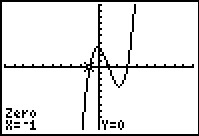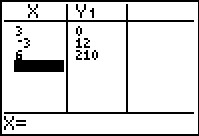# Activities

••• ##### Subject Area

• Math: Algebra II: Polynomials

• ##### Author9-12

60 Minutes

• ##### Device
• TI-83 Plus Family
• TI-84 Plus
• TI-84 Plus Silver Edition
•TI-84 Plus C Silver Edition
•TI-84 Plus CE
• ##### Report an Issue

One of the Many Ways#### Activity Overview

Students graph polynomials to determine the value and number of zeros for a given polynomial.

#### Key Steps

•In this activity, students will graph different degree polynomials equations to determine the value and number of zeros for a given equation. They are to make a conjecture about the number of zeros and the degree of the polynomial.

•In the second part of the activity, students will use the Rational Root Theorem to find the possible zeros of a polynomial and then enter the possible values into a table to determine the actual zeros.